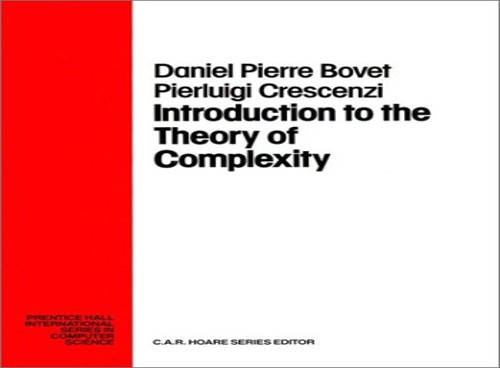# Introduction to the Theory of Complexity by Daniel P. Bovet

In Stock
£34.99
Reviewing in a systematic way the most significant results obtained in the study of computational complexity, this book follows a balanced approach which is partly algorithmic and partly structuralist, assuming a basic knowledge of computational theory, elementary logic and programming techniques.
Only 1 left

## Introduction to the Theory of Complexity Summary

### Introduction to the Theory of Complexity by Daniel P. Bovet

Reviewing in a systematic way the most significant results obtained in the study of computational complexity, this book follows a balanced approach which is partly algorithmic and partly structuralist, assuming a basic knowledge of computational theory, elementary logic and programming techniques. From an algorithmic point of view, Introduction to the Theory of Complexity presents many "natural" problems and limits their complexity by illustrating algorithms which solve them. From a structural point of view, the book concerned with properties of complexity classes, inclusions between classes, implications between several hypotheses about complexity classes, and identification of structural properties of problems that affect their computational complexity. In addition, the book contains a wealth of worked examples and numerous problems.

### Why buy from World of BooksOur excellent value books literally don't cost the earthFree delivery in the UKEvery used book bought is one saved from landfill

1. Mathematical Preliminaries. 2. Elements of Computability Theory. 4. The Class P. 5. The Glass NP. 6. The Complexity of Optiimzation Problems. 7. Beyond NP. 8. Space-Complexity Classes. 9. Probabiillistic. 10. Algorithms and Complexity Classes. 11. Interactivite Proof. 12. Systems. 13. Models of Parallel Computer. 14. Parallel Algorithms.

GOR009930907
Introduction to the Theory of Complexity by Daniel P. Bovet
Daniel P. Bovet
Used - Very Good
Hardback
Pearson Education (US)
1993-11-01
296
0139153802
9780139153808
N/A
Book picture is for illustrative purposes only, actual binding, cover or edition may vary.
This is a used book - there is no escaping the fact it has been read by someone else and it will show signs of wear and previous use. Overall we expect it to be in very good condition, but if you are not entirely satisfied please get in touch with us.